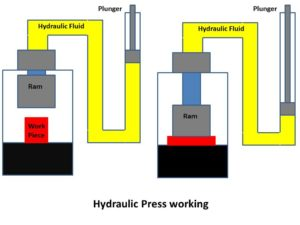# Hydraulic Press : Principle, Construction, Working with Applications

Sharing is Caring :)-

Hydraulic press is a mechanical device which is based on the ‘Pascal’s law’ which states that equal intensity of pressure exerts on all the directions in a closed system. It applicable here in such a way that if there is any pressure change at one point in a closed system then same intensity of pressure will change at other point in the same system.Hydraulic press is able to develop high forces with the application of less effort. There are two main parts in the hydraulic press assembly, one is called as ram and second one is known as plunger. Ram works as output medium where as plunger gives input. In between ram and plunger hydraulic fluid is filled in a closed container which is responsible for the whole operation i.e. force and pressure transmission. In practical situation both plunger and ram have different area. If ‘A’ is the area of ram and ‘a’ is the area of plunger then the mechanical advantage of the system is equal to A/a; which is the ratio of ram area to plunger area because pressure is defined as the force per unit area, if F is the force applied to the plunger and W is the load lift by the ram so according to the Pascal’s law pressure is

P = F/a = W/A     or      W = F (A/a)

Above relations shows that by adjusting the area of the ram and plunger we can able to use it in several applications where we can easily develop high forces by applying only small amount of force.

### Construction and working of Hydrualic Press:

In practical hydraulic press system, generally multiple rams are assembled together. The number of rams used depends upon the working load. In hydraulic press multiple rams of small sizes are preferred instead of a single large size ram to control the thrust forces because it is easy to control the thrust forces on small size as compare to large size. In press assembly one side/table is always fixed while the other is moving by the application of ram force and in between fixed as free side pressing operation is take place. Ram is operated by the hydraulic pressure of fluid. The high pressure liquid is supplied by using pump and hydraulic accumulator. Hydraulic accumulator works as the junction between the pump and the rams. Hydraulic accumulator stores the high pressure liquid when press is at stationary position. Hydraulic press is used where high thrust is required for operation.### Applications:

• The major application of the hydraulic press is in industry where big size metal objects transform into thin sheets by the application of pressure force.
• Sheet metal operations such as drawing, deep drawing, punching and blanking etc
• In crushing of scraps and old vehicles.
• In Packaging industry.

•